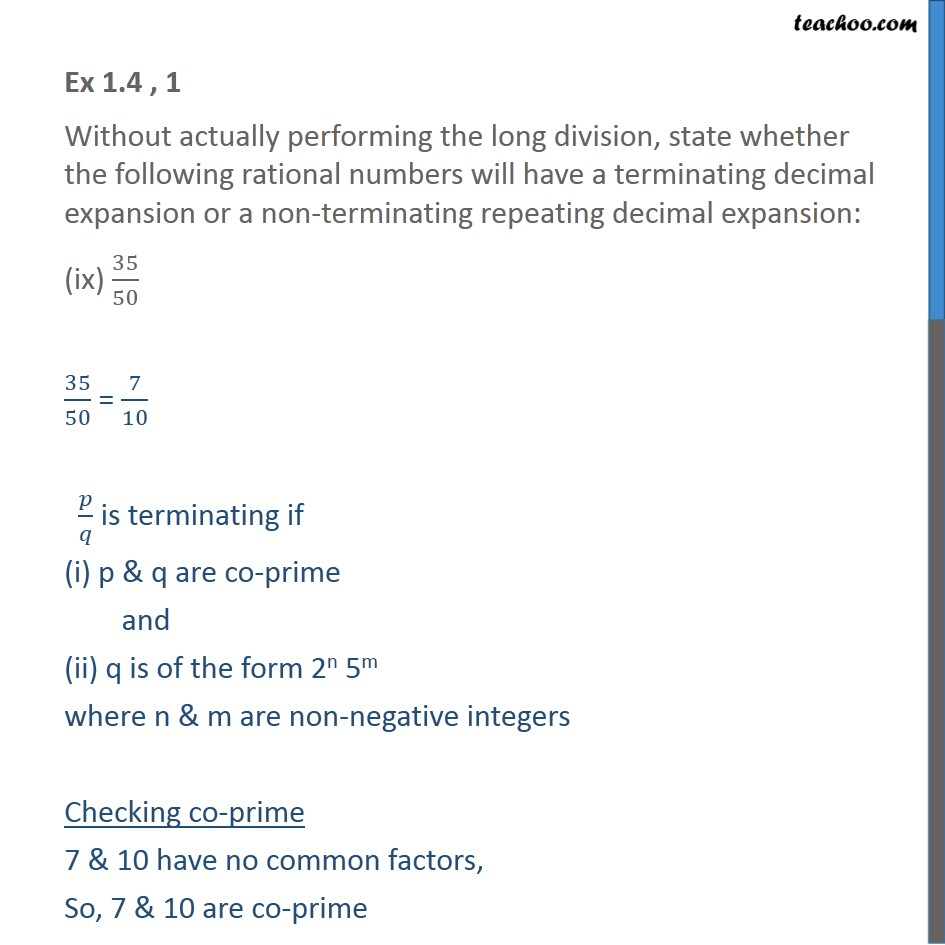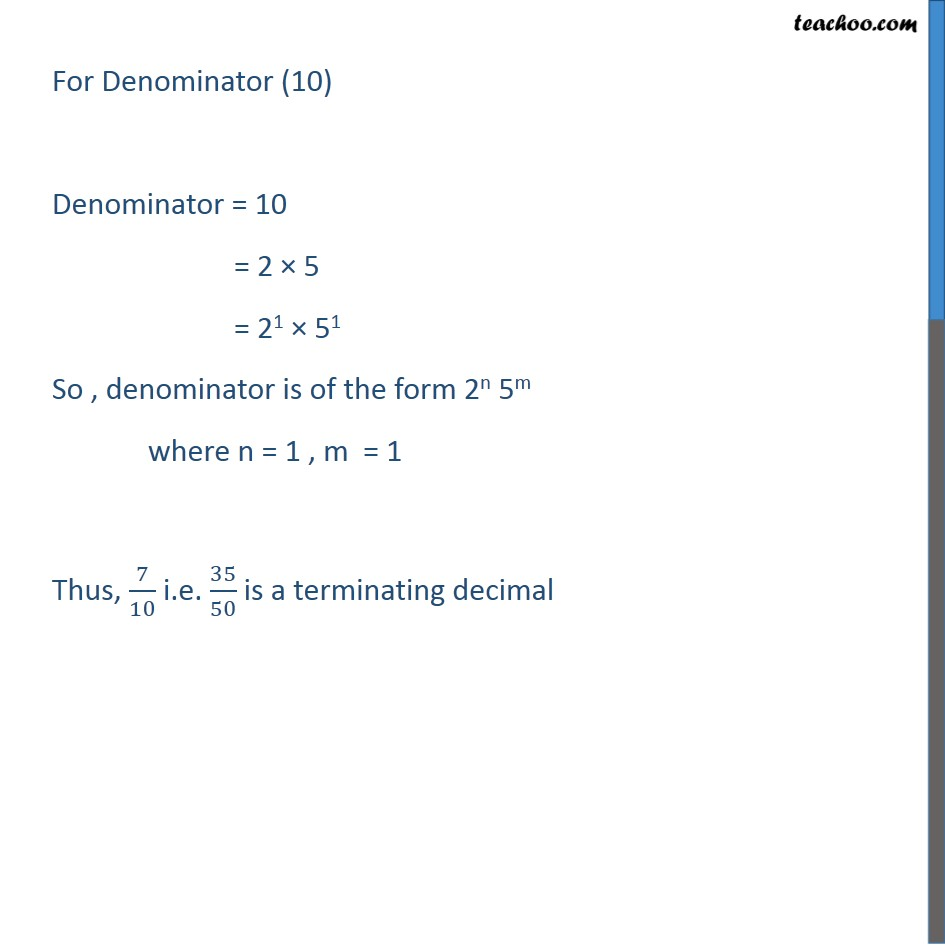Ex 1.4

Chapter 1 Class 10 Real Numbers (Term 1)
Serial order wise### Transcript

Ex 1.4 , 1 Without actually performing the long division, state whether the following rational numbers will have a terminating decimal expansion or a non-terminating repeating decimal expansion: (ix) 35/50 35/50 = 7/10 𝑝/𝑞 is terminating if (i) p & q are co-prime and (ii) q is of the form 2n 5m where n & m are non-negative integers Checking co-prime 7 & 10 have no common factors, So, 7 & 10 are co-prime For Denominator (10) Denominator = 10 = 2 × 5 = 21 × 51 So , denominator is of the form 2n 5m where n = 1 , m = 1 Thus, 7/10 i.e. 35/50 is a terminating decimal